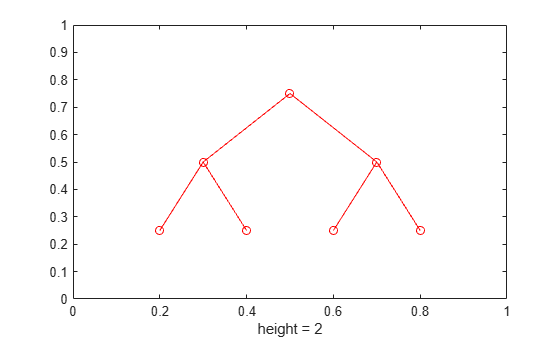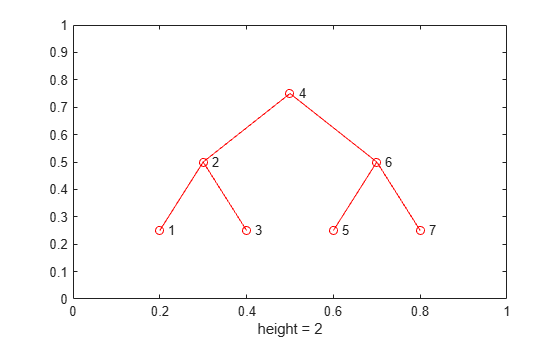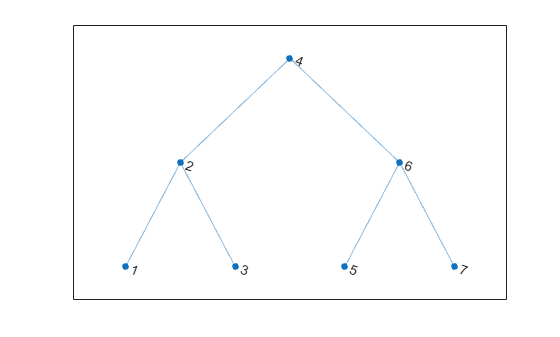# treeplot

Plot picture of tree

## Syntax

``treeplot(p)``
``treeplot(p,NodeSpec,EdgeSpec)``

## Description

example

````treeplot(p)` plots one or more trees specified as a row vector of parent indices. `p(i) = j` indicates that node `j` is a parent of node `i`, and ```p(i) = 0``` indicates that node `i` is a root node.```

example

````treeplot(p,NodeSpec,EdgeSpec)` sets the line style, marker symbol, and color for the nodes and edges in the tree plot. Use `''` to omit the `NodeSpec` or `EdgeSpec` argument.```

## Examples

collapse all

Plot a binary tree with 7 nodes.

Specify a row vector where each element contains the index of its parent node. Specify the root node with a value of `0`. For example, specify the parent of node `1` as `2`, the parent of node `2` as `4`, the parent of node `3` as `2`, and so on.

`p = [2 4 2 0 6 4 6]`
```p = 1×7 2 4 2 0 6 4 6 ```

Plot the binary tree using `treeplot`.

`treeplot(p)`Generate the coordinates of the tree nodes using the `treelayout` function. Add text descriptions to show the node indices in the tree plot. Position the text labels `0.02` units to the right of their corresponding nodes.

```[x,y] = treelayout(p); text(x + 0.02,y,{1,2,3,4,5,6,7})```Another alternative to represent the tree structure is to use a `digraph` object. You can then visualize the structure with `plot`.

Create a `digraph` object using the syntax `digraph(s,t)` that specifies directed graph edges `(s,t)` in pairs. Visualize the tree with `plot`. Suppress the arrows from parent to child nodes by setting the `ShowArrows` option to `false`.

```g = digraph(p(p~=0),find(p)); plot(g,"ShowArrows",false)```Plot two trees. Specify node `1` and node `7` as root nodes by setting their parent indices to `0`. Use blue circles for the nodes, and red-dotted lines with cross markers for the edges.

```p = [0 1 1 2 3 3 0 7 7]; treeplot(p,'bo','rx:')```## Input Arguments

collapse all

Indices of parent nodes, specified as a row vector of positive integers less than or equal to `length(p)`.

• If node `i` is a root node, then specify `p(i)` as `0`.

• If node `i` has a parent node, then specify `p(i)` as the parent of node `i`.

Data Types: `double`

Node marker and color, specified as a character vector or string containing symbols. The symbols can appear in any order. You do not need to specify both marker and color. For example, if you specify the marker and omit the color, then the plot shows only the marker with the default red color.

Example: `'ob'` represents circle markers with blue color

MarkerDescription
`'o'`Circle
`'+'`Plus sign
`'*'`Asterisk
`'.'`Point
`'x'`Cross
`'_'`Horizontal line
`'|'`Vertical line
`'s'`Square
`'d'`Diamond
`'^'`Upward-pointing triangle
`'v'`Downward-pointing triangle
`'>'`Right-pointing triangle
`'<'`Left-pointing triangle
`'p'`Pentagram
`'h'`Hexagram
ColorDescription

`y`

yellow

`m`

magenta

`c`

cyan

`r`

red

`g`

green

`b`

blue

`w`

white

`k`

black

Edge style, marker, and color, specified as a character vector or string containing symbols. The symbols can appear in any order. You do not need to specify all three characteristics (edge style, marker, and color). For example, if you omit the edge style and specify the marker, then the plot shows only the marker and no line.

Example: `'--or'` is a red dashed edge with circle markers

Edge StyleDescription
`-`Solid line
`--`Dashed line
`:`Dotted line
`-.`Dash-dot line
MarkerDescription
`'o'`Circle
`'+'`Plus sign
`'*'`Asterisk
`'.'`Point
`'x'`Cross
`'_'`Horizontal line
`'|'`Vertical line
`'s'`Square
`'d'`Diamond
`'^'`Upward-pointing triangle
`'v'`Downward-pointing triangle
`'>'`Right-pointing triangle
`'<'`Left-pointing triangle
`'p'`Pentagram
`'h'`Hexagram
ColorDescription

`y`

yellow

`m`

magenta

`c`

cyan

`r`

red

`g`

green

`b`

blue

`w`

white

`k`

black

## Version History

Introduced before R2006a